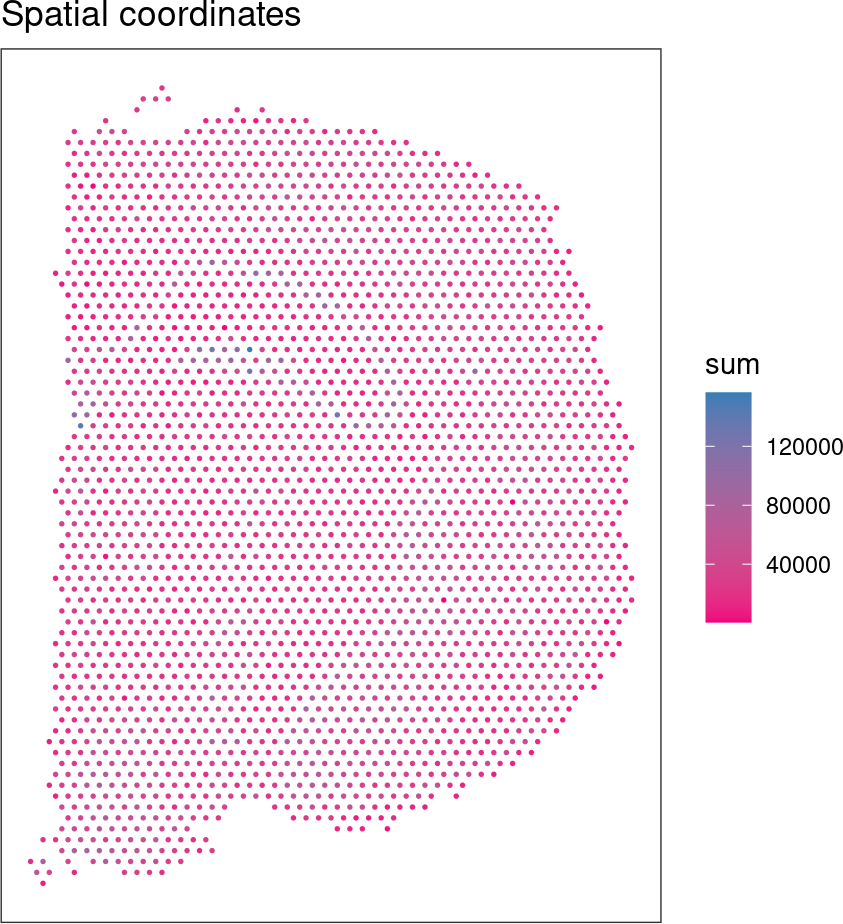# 1 Introduction

The `ggspavis` package contains a set of visualization functions for spatially resolved transcriptomics data, designed to work with the SpatialExperiment Bioconductor object class.

These plotting functions are used in our online book OSTA and other work.

# 2 Examples

Load some example datasets from the STexampleData package and create some example plots.

``````library(SpatialExperiment)
library(STexampleData)``````
``````## Warning: replacing previous import 'utils::findMatches' by
``library(ggspavis)``

## 2.1 10x Genomics Visium: mouse coronal brain section

``````# load data in SpatialExperiment format
spe <- Visium_mouseCoronal()``````
``````# add some values in 'colData' to annotate spots
colData(spe)\$sum <- colSums(counts(spe))

# example plots
plotSpots(spe, annotate = "sum")````````plotVisium(spe, fill = "sum", trans = "log", highlight = "in_tissue")``